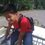# A Trigo Solution

At our Math 17 class, we were asked to solve a trigo problem. The question is,

$\cos \theta+\sin \theta =1$ ,such that $0 \leq \theta < 2\pi$

solve for $\theta$

My Solution:

$\cos \theta+\sin \theta =1$

#### From the eq. $\cos^{2} \theta + \sin^{2} \theta = 1$

$\cos \theta \pm \sqrt{1-\cos^{2} \theta}=1$

$\pm \sqrt{1-\cos^{2} \theta}=1-\cos \theta$

#### Squaring Both Sides,

$1-\cos^{2} \theta =1-2\cos \theta+\cos^{2} \theta$

$0=2\cos^{2} \theta-2\cos \theta$

$\cos^{2} \theta-\cos \theta=0$

$\cos \theta (\cos \theta-1)=0$

$\cos \theta=0$ and $\cos \theta-1=0$

Therefore,

$\theta=90^\circ, 270^\circ$ and $0^\circ$

My Professor's Solution:

$\cos \theta+\sin \theta =1$

#### Squaring Both Sides

$\cos^{2} \theta +2\cos \theta \sin \theta + \sin^{2} \theta = 1$

$2\cos \theta \sin \theta +1=1$

$2\cos \theta \sin \theta =0$

$\cos \theta = 0$ and $\sin \theta =0$

Thus,

$\theta= 90^\circ, 270^\circ, 180^\circ$ and $0^\circ$

She wonders why my answer and her's are different. According to her, my solution is correct but came up with different answers. Could it be that there are some misconceptions? What would be the real answer?Note by Jeffer Dave Cagubcob
7 years ago

This discussion board is a place to discuss our Daily Challenges and the math and science related to those challenges. Explanations are more than just a solution — they should explain the steps and thinking strategies that you used to obtain the solution. Comments should further the discussion of math and science.

When posting on Brilliant:

• Use the emojis to react to an explanation, whether you're congratulating a job well done , or just really confused .
• Ask specific questions about the challenge or the steps in somebody's explanation. Well-posed questions can add a lot to the discussion, but posting "I don't understand!" doesn't help anyone.
• Try to contribute something new to the discussion, whether it is an extension, generalization or other idea related to the challenge.

MarkdownAppears as
*italics* or _italics_ italics
**bold** or __bold__ bold
- bulleted- list
• bulleted
• list
1. numbered2. list
1. numbered
2. list
Note: you must add a full line of space before and after lists for them to show up correctly
paragraph 1paragraph 2

paragraph 1

paragraph 2

[example link](https://brilliant.org)example link
> This is a quote
This is a quote
    # I indented these lines
# 4 spaces, and now they show
# up as a code block.

print "hello world"
# I indented these lines
# 4 spaces, and now they show
# up as a code block.

print "hello world"
MathAppears as
Remember to wrap math in $$ ... $$ or $ ... $ to ensure proper formatting.
2 \times 3 $2 \times 3$
2^{34} $2^{34}$
a_{i-1} $a_{i-1}$
\frac{2}{3} $\frac{2}{3}$
\sqrt{2} $\sqrt{2}$
\sum_{i=1}^3 $\sum_{i=1}^3$
\sin \theta $\sin \theta$
\boxed{123} $\boxed{123}$

Sort by:

Both of your answers are wrong. Both errors come on from "squaring both sides". In your case, the effect of squaring both sides means that when you find $\cos\theta=0$ as a solution, you take both $90^\circ$ and $270^\circ$ as possible answers (since you are allowing $\sin\theta = \pm1$. If you look at the original equation, if $\cos\theta=0$, we must have $\sin\theta=1$, so $270^\circ$ is not an answer.

Your professor is also finding the solutions to the equation $\cos\theta+\sin\theta=-1$, so is getting twice as many answers as there are.

The best solution is: $\begin{array}{rcl} \sin\theta + \cos\theta & = & 1 \\ \tfrac{1}{\sqrt{2}}\sin\theta + \tfrac{1}{\sqrt{2}}\cos\theta & = & \tfrac{1}{\sqrt{2}} \\ \sin(\theta+45^\circ) & = & \sin45^\circ \\ \theta + 45^\circ & = & 45^\circ\,,\,135^\circ \\ \theta & = & 0^\circ\,,\,90^\circ \end{array}$

- 7 years ago

Thanks Mark, I agree with what you said about my Professor's mistake but I don't really get what is my mistake when I square both sides.

- 7 years ago

Your arguement goes: $\begin{array}{rcl} \sin\theta + \cos\theta & = & 1 \\ \sin\theta & = & 1 - \cos\theta \\ \sin^2\theta & = & (1 - \cos\theta)^2 \end{array}$ The third line includes the option that $-\sin\theta = 1 - \cos\theta$. In other words, in addition to solving $\cos\theta+\sin\theta=1$ ($\theta=0^\circ,90^\circ$), you are also solving $\cos\theta-\sin\theta = 1$ ($\theta=0^\circ,270^\circ$).

The problem with squaring both sides is that the argument does not go both ways. While it is true that $a^2=b^2$ whenever $a=b$, it is not always the case that $a=b$ whenever $a^2=b^2$. The method of squaring both sides is only totally safe when both sides of the equation are known to be positive (or both negative). In other words: $\mbox{If } \,a,b \ge 0 \quad \mbox{then} \quad a = b \qquad \Leftrightarrow \qquad a^2 = b^2$

- 7 years ago

Good explanation.

Always remember that when you manipulate equations (squaring, cubing, multiplying, adding, dividing, etc) you risk introducing additional solutions into the system. As such, you have to check that your solutions are indeed solutions, otherwise you will run into errors like claiming $1 = - 1$.

Part of the reason why we don't realize this, is that we mostly deal with simultaneous linear equations, in which the operations that we do (addition of 2 equations, multiplication by a constant, substitution of variables) is well behaved. Outside of that, things could go wrong.

Staff - 7 years ago

Ahh.. Now i get it. So, lesson learned. XD . Thank you so much Mark. Also, I like your strategy in solving that problem.

- 7 years ago

Nice :O I was thinking of making use of the R-formula to approach this question

- 7 years ago

very brilliant solution for mark

- 6 years, 11 months ago

I agree.

- 6 years, 11 months ago